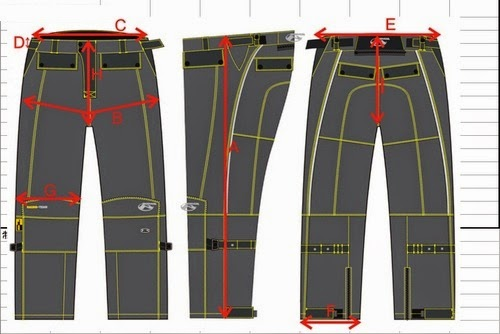# Textile Calculation

Problems and solutions of textile

## Fabric Consumption Formula for Trouser / Pant

Trouser Consumption Formula
Noor Ahmed Raaz
B.Sc. in Apparel Manufacturing
Asst. Merchandiser
Opex Sinha Group, Narayongonj
Email: raju.uttara72@yahoo.com

Trouser consumption calculation
Trouser consumption calculation is widely used in garment industry and specially in apparel merchandising. It is important tusk for merchandiser. If calculation is not correct then company will face to huge loss and reputation also fall.Trouser design
For example:
If you consider,
• Out seam = 42 inch
• In seam = 32”
• Waist = 36”
• Hip = 40”
• Bottom = 20 “
• Fabric width =58”
Mathematical formula to calculate the fabric consumption of trouser in yard is given bellow:

Formula == [ (42” + 2” + 2”) × (Hip/4 + 1.5”) × 4/36”] / 57”

= [ 46” × (40”/4 + 3”) × 4/36”] / 57”

=[ 46” × 13” × 4/36”] / 57”

= 1.165 yd/ per trouser

= 1.165 yd/ per trouser + zipper fly (2) + pocket facing (4) + back pocket bone + belt loop

= 1.165 + 0.058

= 1.21

= 1.21 + 5%

= 1.21 + 0.060

= 1.270 × 12 (per doz)

= 15.24 yard/doz